# BCI-algebra

Algebras introduced by K. Iséki in [a4] as a generalized version of BCK-algebras (cf. BCK-algebra). The latter were developed by Iséki and S. Tannaka in [a6] to generalize the set difference in set theory, and by Y. Imai and Iséki in [a3] as the algebras of certain propositional calculi. It turns out that Abelian groups (cf. Abelian group) are a special case of BCI-algebras. One may take different axiom systems for BCI-algebras, and one such system says that a BCI-algebra is a non-empty setwith a binary relationand a constant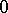satisfying

i);

ii);

iii)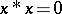;

iv)and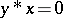imply that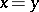;

v)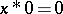implies that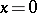. A partial ordermay be defined by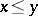if and only if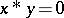. A very useful identity satisfied byis. One can then develop many of the usual algebraic concepts. An ideal is a setwith the properties that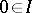and that wheneverand, then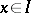. The ideal is implicative ifandimply that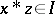. It is known that one always has. An idealis closed if wheneverthen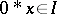. While ideals in general are not subalgebras, closed ideals are. A subalgebra simply means a subset containingand closed underthat is itself a BCI-algebra under.

The subset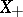of all elements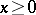forms an ideal, called the-radical of. The algebrais a BCK-algebra if and only if, andis-semi-simple if and only if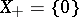. In the latter case,satisfies the identity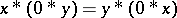for alland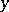. It then follows that one can define an operation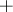onby, and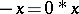. This makesinto an Abelian group withas the identity. Conversely, every Abelian groupcan be given a BCI-algebra structure by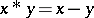. It follows that the category of Abelian groups is equivalent to the subcategory of the category of BCI-algebras formed by the-semi-simple BCI-algebras. Here, a homomorphismfrom one BCI-algebra to another is a function satisfying. In general,always contains a-semi-simple BCI-subalgebra, namely its-semi-simple part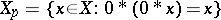. Of course, also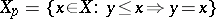, since it can be verified easily that the induced partial order in a-semi-simple BCI-algebra is always trivial. Clearly,is-semi-simple if, andis a BCK-algebra if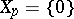. Note that for a-semi-simple BCI-algebra, the closed ideals are precisely the subgroups of the associated Abelian group structure.

Some of the homological algebra properties of BCI-algebras are known. For example, it is known that a BCI-algebra is injective if and only if it is-semi-simple and its associated Abelian group structure is divisible (cf, also Divisible group).

Fuzzy ideals of BCI-algebras are described in [a1] and [a2].

How to Cite This Entry:
BCI-algebra. C.S. Hoo (originator), Encyclopedia of Mathematics. URL: http://encyclopediaofmath.org/index.php?title=BCI-algebra&oldid=12055
This text originally appeared in Encyclopedia of Mathematics - ISBN 1402006098